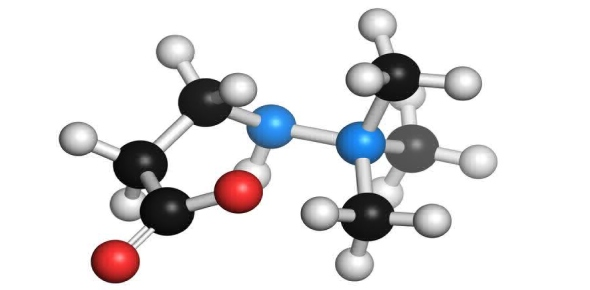What will be the wavelength of the photo emitted when a hydrogen atom - ProProfs DiscussTopicsMoreProductsMore+ Ask Question

# What will be the wavelength of the photo emitted when a hydrogen atom undergoes a transition from n=5 to n=C. (R=1.096776x107 m-1)? Reference : Line spectra from all regions of the electromagnetic spectrum, including the Paschen series of infrared lines for hydrogen, are used by astronomers to identify elements present in the atmospheres of starts.Change Image    Delete

A. 205.1 nm
B. 384.6 nm
C. 683.8 nm
D. 1282 nm
E. >1500 nm

This question is part of electron configuration Test
Asked by Bbrocker, Last updated: Feb 20, 2020

###Request 0FollowShareAnswer AnonymouslyAnswer LaterCopy Link#### k.Paul

K.Paul

To calculate the wavelength, you will need to find the velocity of the wave and divide it by the frequency. Usually waves are measures in meters. So, the velocity is the speed of the wave. Frequency is usually measures in Hertz. This is equivalent to one second. Using the formula, you will then plug in the numbers of the frequency and wavespeed.

Once you calculate this, this will be the answer. Scientific notation should be used in order to make sure the answer is accurate. Since this is a photon, you will need to use another formula which is E = RE x (1/n21 – 1/n22). RE is equal to the Rydberg constant and n1 is the final energy level of the electron and n2 is the initial energy level of the electron.#### John Smith

John Smith1282 nm1Replied on Oct 15, 2017

How did u get thatReplySearch for Google imagesSelect a recommended image
Upload from your computerCancelSearch for Google imagesSelect a recommended image
Upload from your computerCancelSearch for Google imagesSelect a recommended image
Upload from your computerCancel